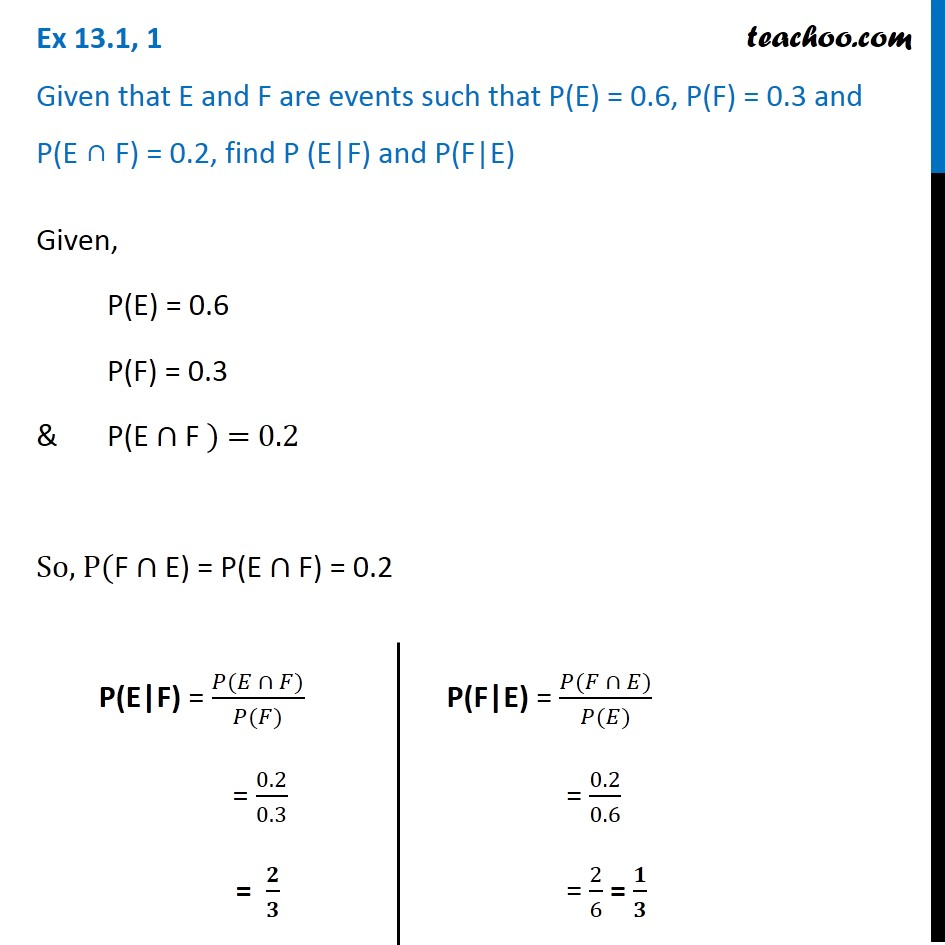1. Chapter 13 Class 12 Probability (Term 2)
2. Serial order wise
3. Ex 13.1

Transcript

Ex 13.1, 1 Given that E and F are events such that P(E) = 0.6, P(F) = 0.3 and P(E ∩ F) = 0.2, find P (E|F) and P(F|E)Given, P(E) = 0.6 P(F) = 0.3 & P(E ∩ F ) = 0.2 So, P(F ∩ E) = P(E ∩ F) = 0.2 P(E|F) = (𝑃(𝐸 ∩ 𝐹))/(𝑃(𝐹)) = 0.2/0.3 = 𝟐/𝟑 P(F|E) = (𝑃(𝐹 ∩ 𝐸))/(𝑃(𝐸)) = 0.2/0.6 = 2/6 = 𝟏/𝟑

Ex 13.1